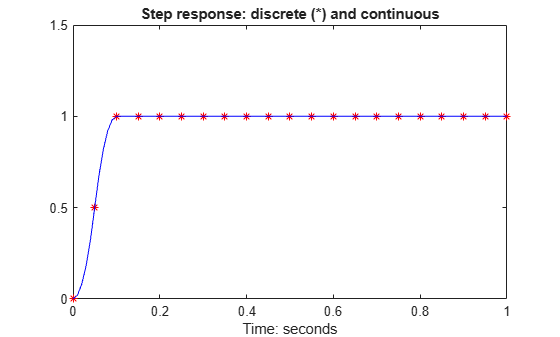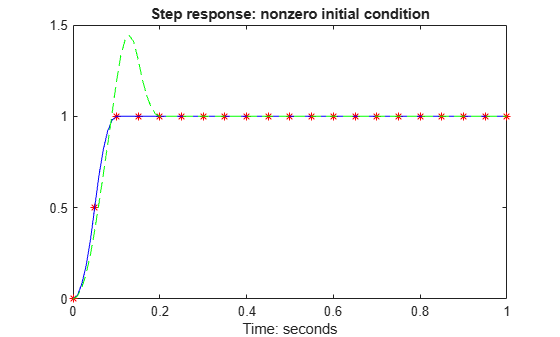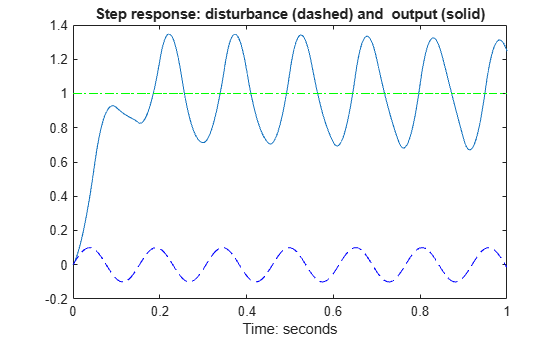# sdlsim

Time response of sampled-data feedback system

## Syntax

```sdlsim(p,k,w,t,tf)
sdlsim(p,k,w,t,tf,x0,z0)
sdlsim(p,k,w,t,tf,x0,z0,int)
[vt,yt,ut,t] = sdlsim(p,k,w,t,tf)
[vt,yt,ut,t] = sdlsim(p,k,w,t,tf,x0,z0,int)
```

## Description

`sdlsim(p,k,w,t,tf) ` plots the time response of the hybrid feedback system. `lft(p,k)`, is forced by the continuous input signal described by `w` and `t` (values and times, as in `lsim`). `p` must be a continuous-time LTI system, and `k` must be discrete-time LTI system with a specified sample time (the unspecified sample time –1 is not allowed). The final time is specified with `tf`.

`sdlsim(p,k,w,t,tf,x0,z0) ` specifies the initial state vector `x0` of `p`, and `z0` of `k`, at time` t(1)`.

`sdlsim(p,k,w,t,tf,x0,z0,int) ` specifies the continuous-time integration step size `int`. `sdlsim` forces `int = (k.Ts)/N int` where N>4 is an integer. If any of these optional arguments is omitted, or passed as empty matrices, then default values are used. The default value for `x0` and `z0` is zero. Nonzero initial conditions are allowed for `p` (and/or` k`) only if` p` (and/or `k`) is an `ss` object.

If `p` and/or `k` is an LTI array with consistent array dimensions, then the time simulation is performed pointwise across the array dimensions.

`[vt,yt,ut,t] = sdlsim(p,k,w,t,tf) ` computes the continuous-time response of the hybrid feedback system `lft(p,k)` forced by the continuous input signal defined by `w` and `t` (values and times, as in `lsim`). `p` must be a continuous-time system, and `k` must be discrete-time, with a specified sample time (the unspecified sample time –1 is not allowed). The final time is specified with `tf`. The outputs `vt, yt` and `ut` are 2-by-1 cell arrays: in each the first entry is a time vector, and the second entry is the signal values. Stored in this manner, the signal `vt` is plotted by using one of the following commands:

```plot(vt{1},vt{2}) plot(vt{:}) ```

Signals `yt` and `ut` are respectively the input to `k` and output of `k`.

If `p` and/or` k` are LTI arrays with consistent array dimensions, then the time simulation is performed pointwise across the array dimensions. The outputs are 2-by-1-by-array dimension cell arrays. All responses can be plotted simultaneously, for example, `plot(vt)`.

`[vt,yt,ut,t] = sdlsim(p,k,w,t,tf,x0,z0,int)` The optional arguments are `int` (integration step size), `x0` (initial condition for `p`), and `z0` (initial condition for `k`). `sdlsim` forces `int = (k.Ts)/N`, where N>4 is an integer. If any of these arguments is omitted, or passed as empty matrices, then default values are used. The default value for `x0` and `z0` is zero. Nonzero initial conditions are allowed for `p` (and/or `k`) only if `p` (and/or `k`) is an `ss` object.

## Examples

collapse all

To illustrate the use of `sdlsim`, consider the application of a discrete controller to a plant with an integrator and near integrator. A continuous plant and a discrete controller are created. A sample-and-hold equivalent of the plant is formed and the discrete closed-loop system is calculated. Simulating this gives the system response at the sample points. `sdlsim` is then used to calculate the intersample behavior.

```P = tf(1,[1, 1e-5,0]); T = 1.0/20; C = ss([-1.5 T/4; -2/T -.5],[ .5 2;1/T 1/T],... [-1/T^2 -1.5/T], [1/T^2 0],T); Pd = c2d(P,T,'zoh');```

Use `connect` to construct the interconnected feedback system.

```C.InputName = {'ref','y'}; C.OutputName = 'u'; Pd.Inputname = 'u'; Pd.OutputName = 'y'; dclp = connect(C,Pd,'ref','y');```

Use `step` to simulate the digital step response.

`[yd,td] = step(dclp,20*T);`

Set up the continuous interconnection and calculate the sampled data response with `sdlsim`.

```M = [0,1;1,0;0,1]*blkdiag(1,P); t = [0:.01:1]'; u = ones(size(t)); y1 = sdlsim(M,C,u,t); plot(td,yd,'r*',y1{:},'b-') axis([0,1,0,1.5]) xlabel('Time: seconds') title('Step response: discrete (*) and continuous')```You can see the effect of a nonzero initial condition in the continuous-time system. Note how examining the system at only the sample points will underestimate the amplitude of the overshoot.

```y2 = sdlsim(M,C,u,t,1,0,[0.25;0]); plot(td,yd,'r*',y1{:},'b-',y2{:},'g--') axis([0,1,0,1.5]) xlabel('Time: seconds') title('Step response: nonzero initial condition')```Finally, you can examine the effect of a sinusoidal disturbance at the continuous-time plant output. This controller is not designed to reject such a disturbance and the system does not contain antialiasing filters. Simulating the effect of antialiasing filters is easily accomplished by including them in the continuous interconnection structure.

```M2 = [0,1,1;1,0,0;0,1,1]*blkdiag(1,1,P); t = [0:.001:1]'; dist = 0.1*sin(41*t); u = ones(size(t)); [y3,meas,act] = sdlsim(M2,C,[u dist],t,1); plot(y3{:},'-',t,dist,'b--',t,u,'g-.') xlabel('Time: seconds') title('Step response: disturbance (dashed) and output (solid)')```## Algorithms

`sdlsim` oversamples the continuous-time, N times the sample rate of the controller k.